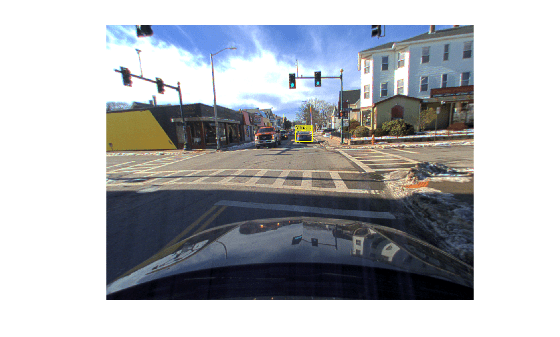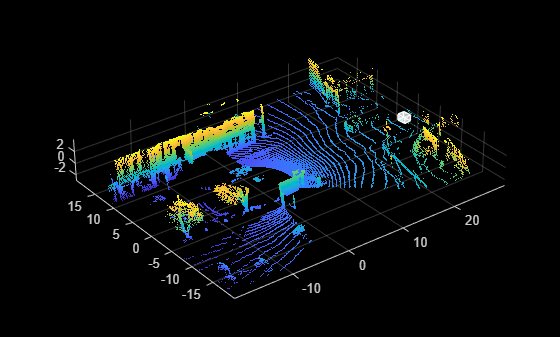# bboxCameraToLidar

Estimate 3-D bounding boxes in point cloud from 2-D bounding boxes in image

Since R2020b

## Syntax

``bboxesLidar = bboxCameraToLidar(bboxesCamera,ptCloudIn,intrinsics,tform)``
``[bboxesLidar,indices] = bboxCameraToLidar(___)``
``[bboxesLidar,indices,boxesUsed] = bboxCameraToLidar(___)``
``[___] = bboxCameraToLidar(___,Name,Value)``

## Description

````bboxesLidar = bboxCameraToLidar(bboxesCamera,ptCloudIn,intrinsics,tform)` estimates 3-D bounding boxes in a point cloud frame, `ptCloudIn`, from 2-D bounding boxes in an image, `bboxesCamera`. The function uses camera intrinsic parameters, `intrinsics`, and a camera to lidar transformation matrix, `tform`, to estimate the 3-D bounding boxes, `bboxesLidar`.```
````[bboxesLidar,indices] = bboxCameraToLidar(___)` returns the indices of the point cloud points that are inside the 3-D bounding boxes using the input arguments from the previous syntax.```
````[bboxesLidar,indices,boxesUsed] = bboxCameraToLidar(___)` indicates for which of the specified 2-D bounding boxes the function detected a corresponding 3-D bounding box in the point cloud.```

example

````[___] = bboxCameraToLidar(___,Name,Value)` specifies options using one or more name-value arguments in addition to any of the argument combinations in previous syntaxes. For example, `'ClusterThreshold',0.5` sets the Euclidean distance threshold for differentiating point cloud clusters to 0.5 world units.```

## Examples

collapse all

Load ground truth data from a MAT-file into the workspace. Extract the image, point cloud data, and camera intrinsic parameters from the ground truth data.

```dataPath = fullfile(toolboxdir('lidar'),'lidardata','lcc','bboxGT.mat'); gt = load(dataPath); im = gt.im; pc = gt.pc; intrinsics = gt.cameraParams;```

Extract the camera to lidar transformation matrix from the ground truth data.

`tform = gt.camToLidar;`

Extract the 2-D bounding box information.

`bboxImage = gt.box;`

Display the 2-D bounding box overlaid on the image.

```annotatedImage = insertObjectAnnotation(im,'Rectangle',bboxImage,'Vehicle'); figure imshow(annotatedImage)```Estimate the bounding box in the point cloud.

```[bboxLidar,indices] = ... bboxCameraToLidar(bboxImage,pc,intrinsics,tform,'ClusterThreshold',1);```

Display the 3-D bounding box overlaid on the point cloud.

```figure pcshow(pc) xlim([0 50]) ylim([0 20]) showShape('cuboid',bboxLidar,'Opacity',0.5,'Color','green')```## Input Arguments

collapse all

2-D bounding boxes in the camera frame, specified as an M-by-4 matrix of real values. Each row of the matrix contains the location and size of a rectangular bounding box in the form [x y width height]. The x and y elements specify the x and y coordinates, respectively, for the upper-left corner of the rectangle. The width and height elements specify the size of the rectangle. M is the number of bounding boxes.

Note

The function assumes that the image data that corresponds to the 2-D bounding boxes and the point cloud data are time synchronized.

Data Types: `single` | `double`

Point cloud, specified as a `pointCloud` object.

Note

The function assumes that the point cloud is in the vehicle coordinate system, where the x-axis points forward from the ego vehicle.

Camera intrinsic parameters, specified as a `cameraIntrinsics` object.

Camera to lidar rigid transformation, specified as a `rigidtform3d` object.

### Name-Value Arguments

Specify optional pairs of arguments as `Name1=Value1,...,NameN=ValueN`, where `Name` is the argument name and `Value` is the corresponding value. Name-value arguments must appear after other arguments, but the order of the pairs does not matter.

Example: `ClusterThreshold=0.5` sets the Euclidean distance threshold for differentiating point cloud clusters to 0.5 world units.

Before R2021a, use commas to separate each name and value, and enclose `Name` in quotes.

Example: `'ClusterThreshold',0.5` sets the Euclidean distance threshold for differentiating point cloud clusters to 0.5 world units.

Clustering threshold for two adjacent points, specified as a positive scalar. The clustering process is based on the Euclidean distance between two adjacent points. If the distance between two adjacent points is less than the specified clustering threshold, then the points belong to the same cluster. If the function returns a 3-D bounding box that is smaller than expected, try specifying a higher `'ClusterThreshold'` value.

Data Types: `single` | `double`

Range of detection from lidar sensor, specified as a two-element vector of real values in the range (`0, Inf`]. The first element of the vector specifies the shortest distance from the sensor at which to search for bounding boxes, and the second element specifies the distance at which the function stops searching. The value of Inf indicates the outermost points of the point cloud.

The first element must be smaller than the second element. Specify both in world units.

Data Types: `single` | `double`

## Output Arguments

collapse all

3-D bounding boxes in the lidar frame, returned as an N-by-9 matrix of real values. N is the number of detected 3-D bounding boxes. Each row of the matrix has the form [xctr yctr zctr xlen ylen zlen xrot yrot zrot].

• xctr, yctr, and zctr — These values specify the x-, y-, and z-axis coordinates, respectively, of the center of the cuboid bounding box.

• xlen, ylen, and zlen — These values specify the length of the cuboid along the x-, y-, and z-axis, respectively, before it is rotated.

• xrot, yrot, and zrot — These values specify the rotation angles of the cuboid around the x-, y-, and z-axis, respectively. These angles are clockwise-positive when looking in the forward direction of their corresponding axes.

This figure shows how these values determine the position of a cuboid.Data Types: `single` | `double`

Indices of the points inside the 3-D bounding boxes, returned as a column vector or an N-element cell array.

If the function detects only one 3-D bounding box in the point cloud, it returns a column vector. Each element of the vector is the point cloud index of a point detected in the 3-D bounding box.

If the function detects multiple 3-D bounding boxes, it returns an N-element cell array. N is the number of 3-D bounding boxes detected in the point cloud, and each element of the cell array contains the point cloud indices of the points detected in the corresponding 3-D bounding box.

Data Types: `single` | `double`

Bounding box detection flag, returned as an M-element row vector of logicals. M is the number of input 2-D bounding boxes. If the function detects a corresponding 3-D bounding box in the point cloud, then it returns a value of `true` for that input 2-D bounding box. If the function does not detect a corresponding 3-D bounding box, then it returns a value of `false`.

Data Types: `logical`

## Version History

Introduced in R2020b

expand all